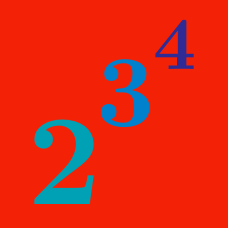Algebra

# Rules of Exponents: Same Base

True or False:

The product rule states that to multiply two exponents with the same base, we keep the base and multiply the powers. For example,

$2 ^ 2 \times 2 ^ 3 = 2 ^ { 2 \times 3 } .$

Simplify $\large x^8 \div x^2.$

Which of the following is equivalent to

$\large 2^{22} - 2^{21} ?$

Suppose that

$\Large x^{\color{#D61F06} {A}}x^{\frac{1}{2}}x^{\frac{1}{4}}x^{\frac{1}{8}}=x.$

What is the value of $\color{#D61F06} {A}$?

Simplify $\large (-x)^2 \times (-x)^4.$

×